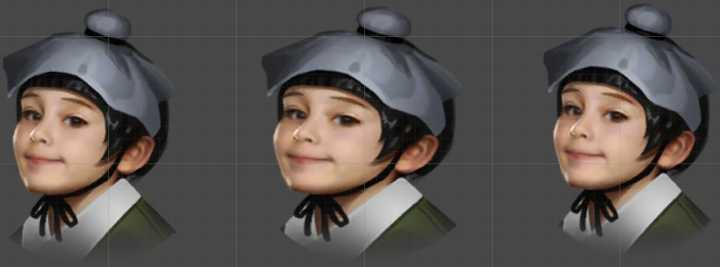Unity里提供了不少功能都有种“磨人的小妖精”的感觉——在你做Demo或者UI需要效果的时候，它扭着妖娆的腰，唱着诱人的歌吸引着你，“来吧~来享用我吧~你可以快速地实现美美的效果，而简单方便，毫无痛楚。”而直到Profiling的时候才发现，正是这些小妖精默默地榨干了你的CPU/GPU/带宽，让你的游戏在设备上变得一步三卡……

## 1. 已有方案对比

``````_StencilComp("Stencil Comparison", Float) = 8
_Stencil("Stencil ID", Float) = 0
_StencilOp("Stencil Operation", Float) = 0

Stencil
{
Ref[_Stencil]
Comp[_StencilComp]
Pass[_StencilOp]
}``````
``````//vert中
OUT.vertex = UnityObjectToClipPos(OUT.worldPosition);
//frag中
color.a *= UnityGet2DClipping(IN.worldPosition.xy, _ClipRect);
#ifdef UNITY_UI_ALPHACLIP
clip(color.a - 0.001);
#endif``````

1. Draw Call，Mask要单独一个Draw call来绘制，额外增加Draw Call，然后它还可能会打断ugui合并draw call的过程，额外增加一些draw call；

Rect Mask 2D的局限性比较明显，只支持矩形，然后因为用的是clip所以没有overdraw的过程，drawcall也友好一些，我猜是ugui里直接处理成参数 float4 _ClipRect 传递给所有孩子节点。

## 3. 基于网格修改的方案

``````using UnityEngine;
using UnityEngine.UI;
using UnityEngine.Sprites;

/// <summary>
/// 添加上方下圆的支持。
/// </summary>
[RequireComponent(typeof(Image))]
public class CircleImage : BaseMeshEffect
{
[Tooltip("圆形或扇形填充比例")]
[Range(0, 1)]
public float fillPercent = 1f;
[Tooltip("圆形")]
[Range(3, 60)]
public int segements = 30;
[Tooltip("是否上方下圆")]
public bool IsTopRect = false;

protected Image image;

private float uvCenterX = 0;
private float uvCenterY = 0;
private float uvScaleX = 0;
private float uvScaleY = 0;

private float vertexCenterX = 0;
private float vertexCenterY = 0;

// Use this for initialization
protected override void Awake ()
{
image = this.GetComponent<Image>();
}

private void AddVertex(VertexHelper vh, float x, float y)
{
UIVertex uiVertex = new UIVertex();
uiVertex.color = image.color;
uiVertex.position.x = vertexCenterX + x;
uiVertex.position.y = vertexCenterY + y;
uiVertex.uv0 = new Vector2(x * uvScaleX + uvCenterX, y * uvScaleY + uvCenterY);
}

public override void ModifyMesh(VertexHelper vh)
{
if (!IsActive())
return;
vh.Clear();

float degreeDelta = (float)(2 * Mathf.PI / segements);
float tempFillPercent = IsTopRect ? 0.5f : fillPercent;

int curSegements = (int)(segements * tempFillPercent);
//为了保证上方是方形时半圆完整，强制增加一个单位弧度的顶点数量
if (IsTopRect)
{
curSegements += 1;
}

float tw = image.rectTransform.rect.width;
float th = image.rectTransform.rect.height;

float outerRadius = 0.5f * tw;

vertexCenterX = (0.5f - image.rectTransform.pivot.x) * tw;
vertexCenterY = (0.5f - image.rectTransform.pivot.y) * th;

Vector4 uv = image.overrideSprite != null ? DataUtility.GetOuterUV(image.overrideSprite) : Vector4.zero;

uvCenterX = (uv.x + uv.z) * 0.5f;
uvCenterY = (uv.y + uv.w) * 0.5f;
uvScaleX = (uv.z - uv.x) / tw;
uvScaleY = (uv.w - uv.y) / th;

float curDegree = 0;
int verticeCount;
int triangleCount;

verticeCount = curSegements + 1;

//添加圆弧顶点
for (int i = 1; i < verticeCount; i++)
{
float cosA = Mathf.Cos(curDegree);
float sinA = Mathf.Sin(curDegree);
curDegree -= degreeDelta;

}

//如果上方下园则添加方形的顶点
if (IsTopRect)
{
curSegements += 2;
verticeCount += 2;

}

triangleCount = curSegements*3;
for (int i = 0, vIdx = 1; i < triangleCount - 3; i += 3, vIdx++)
{
}
if (fillPercent == 1 || IsTopRect)
{
//首尾顶点相连
}
}

}
``````

``````//Github上的原始代码
float tw = image.rectTransform.rect.width;
float th = image.rectTransform.rect.height;
float outerRadius = image.rectTransform.pivot.x * tw;
``````

## 4. 基于uv1的修改方案

``````using UnityEngine;
using UnityEngine.UI;
using UnityEngine.Sprites;

/// <summary>
/// 支持共用材质，从而支持合批。
/// </summary>
[RequireComponent(typeof(Image))]
{
protected Image image;

protected override void Awake ()
{
image = this.GetComponent<Image>();
}

public override void ModifyMesh(VertexHelper vh)
{
if (!IsActive())
return;

Vector4 uv = image.overrideSprite != null ? DataUtility.GetOuterUV(image.overrideSprite) : Vector4.zero;
float uvWidth = uv.z - uv.x;
float uvHeight = uv.w - uv.y;
if (uvWidth == 0 || uvHeight == 0)
{
return;
}

int vertCount = vh.currentVertCount;
var vert = new UIVertex();
for (int i = 0; i < vertCount; ++i)
{
vh.PopulateUIVertex(ref vert, i);

vert.uv1.x = (vert.uv0.x - uv.x) / uvWidth;
vert.uv1.y = (vert.uv0.y - uv.y) / uvHeight;
vh.SetUIVertex(vert, i);
}
}
}
``````

``````fixed4 frag(v2f IN) : SV_Target
{
half4 color = (tex2D(_MainTex, IN.texcoord) + _TextureSampleAdd) * IN.color;
//这里其实使用rgb通道中的一个就够了

color.a *= UnityGet2DClipping(IN.worldPosition.xy, _ClipRect);
#ifdef UNITY_UI_ALPHACLIP
clip(color.a - 0.001);
#endif

return color;
}``````

## 6. 补

``````[AddComponentMenu("UI/Custom/Maskable Image")]
{
private static readonly Vector4 s_DefaultTangent = new Vector4(1.0f, 0.0f, 0.0f, -1.0f);
private static readonly Vector3 s_DefaultNormal = Vector3.back;

protected override void GenerateSimpleSprite(VertexHelper vh, bool lPreserveAspect)
{
Vector4 v = GetDrawingDimensions(lPreserveAspect);
var uv = (overrideSprite != null) ? DataUtility.GetOuterUV(overrideSprite) : Vector4.zero;

float uvWidth = uv.z - uv.x;
float uvHeight = uv.w - uv.y;
bool setUV1 = !(uvWidth == 0 || uvHeight == 0);

var color32 = color;
vh.Clear();
vh.AddVert(new Vector3(v.x, v.y), color32, new Vector2(uv.x, uv.y), setUV1 ? new Vector2((uv.x - uv.x) / uvWidth, (uv.y - uv.y) / uvHeight) : Vector2.zero, s_DefaultNormal, s_DefaultTangent);
vh.AddVert(new Vector3(v.x, v.w), color32, new Vector2(uv.x, uv.w), setUV1 ? new Vector2((uv.x - uv.x) / uvWidth, (uv.w - uv.y) / uvHeight) : Vector2.zero, s_DefaultNormal, s_DefaultTangent);
vh.AddVert(new Vector3(v.z, v.w), color32, new Vector2(uv.z, uv.w), setUV1 ? new Vector2((uv.z - uv.x) / uvWidth, (uv.w - uv.y) / uvHeight) : Vector2.zero, s_DefaultNormal, s_DefaultTangent);
vh.AddVert(new Vector3(v.z, v.y), color32, new Vector2(uv.z, uv.y), setUV1 ? new Vector2((uv.z - uv.x) / uvWidth, (uv.y - uv.y) / uvHeight) : Vector2.zero, s_DefaultNormal, s_DefaultTangent);

}
}
``````

ThorImage是我拷贝的Image.cs，开放了部分接口，比如这里需要的GetDrawingDimensions，还有让GenerateSimpleSprite可以被override，需要的自己弄一下就好。

PS：如果你有更好的方法，欢迎反馈给我~~

（2018年1月2日于杭州滨江海创园）